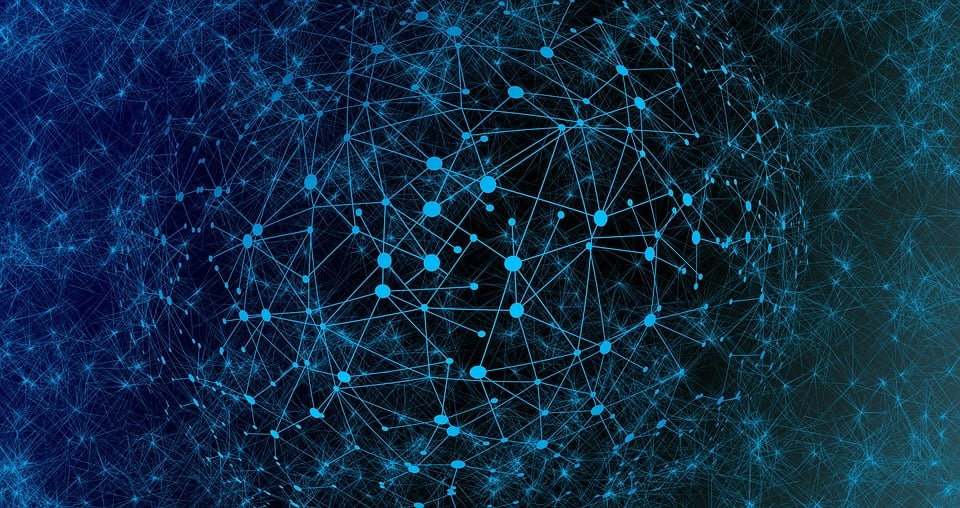I am an associate professor at Twente Universty. I work at the intersection of probability theory, graph theory and stochastic networks, with an emphasis on asymptotic analysis, stochastic process limits, and randomized algorithms. Problems I investigate are inspired by applications in network science, physics and computer science. Some of my research interests are:Epidemic and percolation processes on networks have many applications, ranging from virus spreading to internet outages. I am interested in analyzing epidemic or percolation processes on random graph models: null models for real-world networks. How do communities, small groups of densely connected nodes influence the spread of an epidemic? And what happens at the threshold where a giant outbreak emerges?

### Network motifs

Small subgraphs that occur frequently in a network data set can reveal interesting properties of the underlying data. I am interested in the occurrence of these small subgraphs in random graph models. How frequently do subgraphs appear in random graph models? And where in the graph can they be found? Interestingly, in many random graph models, subgraphs concentrate on a small set of vertices of specific degrees. These vertices can be found by solving optimization problems.

### Asymptotic network properties

Real-world networks are often very large. I am interested in investigating what happens to structural network properties in the large-network limit, such as degree-degree correlations and clustering.

### Geometric networks

In most networks, we can interpret the vertices as lyng in a geometric space. For example, internet users may have a two-dimensional position. But in social media networks, you can interpret the position of a person as its interests, location and so on. In most applications, vertices that are close, are more likely to connect. I am interested in investigating such geometric networks from a mathematical point of view. Can we quantify the robustness of the internet? And how can we get the geometric coordinates from the network structure?